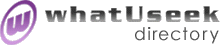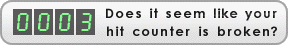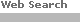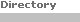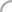SearchThe Directory The Webfor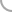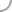Games_and_PuzzlesNew! Submit a site

Categories:
 Mathematical Recreations *(369)whatUseek Collection Sites (submit a site ):

Give your site great placement in this category in as little as two business days!

whatUseek Directory Site Listings:

A+ Math - Interactive math help for K-12 students with flashcards, Math Wordfind, Homework Helper and worksheets.

Brain Teasers and Mathematical Puzzles - Interactive brain teasers to challenge your logical and mathematical skills. Immediate scoring of answers provided with dynamic explanations.

Colorful Mathematics - Educational software presents advanced mathematical concepts to K-12 students in a game-oriented approach, using coloring and drawing techniques to illustrate state of the art mathematical concepts from graph theory and computer science.

Interactive Mathematics Miscellany and Puzzles - Java-based math resource including articles, puzzles and links.

Logicville - Logicville is a website collection of mathematical games, puzzles, and brain teasers, including anagrams, cryptograms, cryptarithms or alphametics, word puzzles, logic games, doublets, tangrams, chess problems, and many educational and math problems.

Macalester College Problem of the Week - Weekly math problem and archive from Professor Stan Wagon for first-year college students presented by the Math Forum.

MatheMagic - Puzzles, jokes and articles.

MatheMajik - Indian site featuring calculation tricks with numbers.

Mathwizz - Interactive math problems for abilities ranging from middle school to university.

Middle School Problem of the Week - A project from the Math Forum presenting a weekly interactive math challenge to accumulate points for bronze, silver and gold prizes. Individual or class participation.

Mr Titen's More Than Math - Mathematics teacher provides puzzles for students.

Problem of the Week - Contest site for all ages with a calculator prize for the winner of each of five weekly challenges. Exclusive prizes for below grade 6 and grades 6-12.

Puzzles from Maths For Fun - Free samples on site updated every Sunday. Cross numbers targeted on specific arithmetical processes, ideal for classroom use (Printable). Our competition page has puzzles taken from PuzzleFrenzy.

Pythabacus - Description and methods of using the Pythagorean Abacus to teach arithmetic.

Quandaries and Queries - Forum for submission of school math problems for solution by math experts.

Quia! Mathematics Activities - Educational activities for teaching mathematics including concentration, matching, flash cards, word search puzzles, and quizzes.

Shack's Math Problems - Over one hundred math problems ranging from basic math to differential equations.

Teaching Kids Math Playing Fun Educational Games - Online games for ages 0-14 with links to free resources.

The Math Forum - Comprehensive online resource for educators and students.

[ 1 2 ]Help build the largest human-edited directory on the web.About   Help   Content Filter   Terms   Privacy Policy© 2018 whatUseek